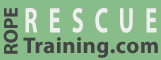# COD Forces

When you pull on a rope that is coming from a load, you are actually subtracting the forces that you are pulling from the forces on the anchor.

For example, in this 3:1 system with a 100 kg load where we are pulling 33.3 kg, the net force on our anchor is actually 66.7 kg (i.e., the 100 kg load minus the 33.3 kg we are pulling).

If you use the same 3:1 system but add a change of direction pulley, you are adding the forces that you are pulling to the forces on the anchor. In this case, the anchor would be holding a total of 133.3 kg (i.e., the 100 kg from the load plus the 33.3 kg that you are pulling).

This is probably immaterial, because your anchor should be plenty-strong to support an increase of 33%.

However, if the change of direction pulley comes before your mechanical advantage system, as shown in this system, the forces on the anchor are doubled. In this example, the anchor receives 100 kg from the load and 100 kg from the system. Doubling the forces on an anchor might be material. (You could decrease the forces on the COD pulley in this system by increasing the angle between the two ropes.)

Working with PowerPoint?
Let vRigger help.